Decrease by a constant factor Decrease by a

• Slides: 11Decrease by a constant factor Decrease by a variable amount A FEW LEFTOVER DECREASE-ANDCONQUER ALGORITHMSDecrease by a Constant Factor • Examples that we have already seen: – Binary Search – Exponentiation (ordinary and modular) by repeated squaring – Multiplication à la Russe (The Dasgupta book that I often used for the first part of the course calls it "European" instead of "Russian") • Example 11 13 5 26 2 52 1 104 143 Then strike out any rows whose first number is even, and add up the remaining numbers in the second column.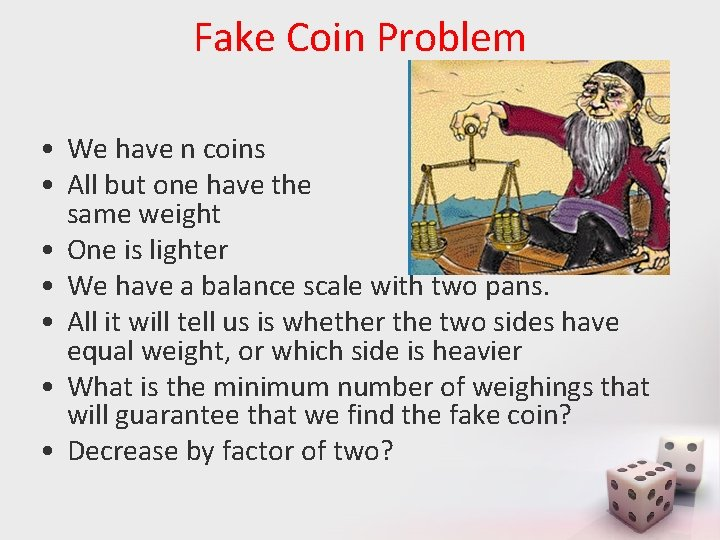Fake Coin Problem • We have n coins • All but one have the same weight • One is lighter • We have a balance scale with two pans. • All it will tell us is whether the two sides have equal weight, or which side is heavier • What is the minimum number of weighings that will guarantee that we find the fake coin? • Decrease by factor of two?Decrease by a variable amount • Search in a Binary Search Tree • Interpolation Search – See Levitin, pp 190 -191 – Also Weiss, Section 5. 6. 3Median finding • Find the kth element of an (unordered) list of n elements • Start with quicksort's partition method • Informal analysis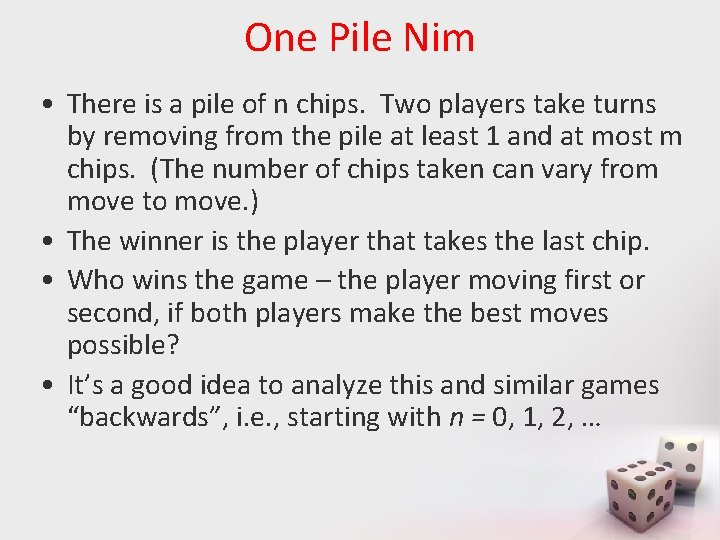One Pile Nim • There is a pile of n chips. Two players take turns by removing from the pile at least 1 and at most m chips. (The number of chips taken can vary from move to move. ) • The winner is the player that takes the last chip. • Who wins the game – the player moving first or second, if both players make the best moves possible? • It’s a good idea to analyze this and similar games “backwards”, i. e. , starting with n = 0, 1, 2, …Graph of One-Pile Nim with m = 4 • Vertex numbers indicate n, the number of chips in the pile. The losing position for the player to move are circled. Only winning moves from a winning position are shown. • Generalization: The player moving first wins iff n is not a multiple of 5 (more generally, m+1); – The winning move is to take n mod 5 (n mod (m+1)) chips on every move.Multi-Pile Nim • There are multiple piles of chips. Two players take turns by removing from any single pile at least 1 and at most all of that pile's chips. (The number of chips taken can vary from move to move) • The winner is the player that takes the last chip. • What is the winning strategy for 2 -pile Nim? • For the general case, consider the "Nim sum", x y, which is the integer obtained by bitwise XOR of corresponding bits of two non-negative integers x and y. What is 6 3?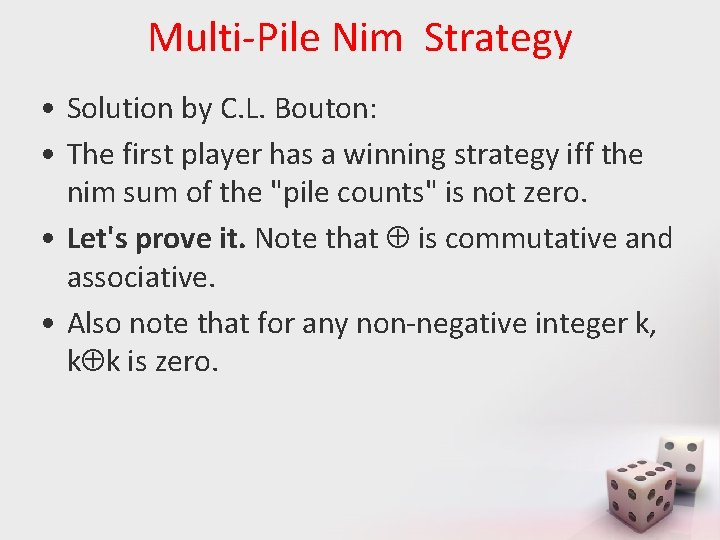Multi-Pile Nim Strategy • Solution by C. L. Bouton: • The first player has a winning strategy iff the nim sum of the "pile counts" is not zero. • Let's prove it. Note that is commutative and associative. • Also note that for any non-negative integer k, k k is zero.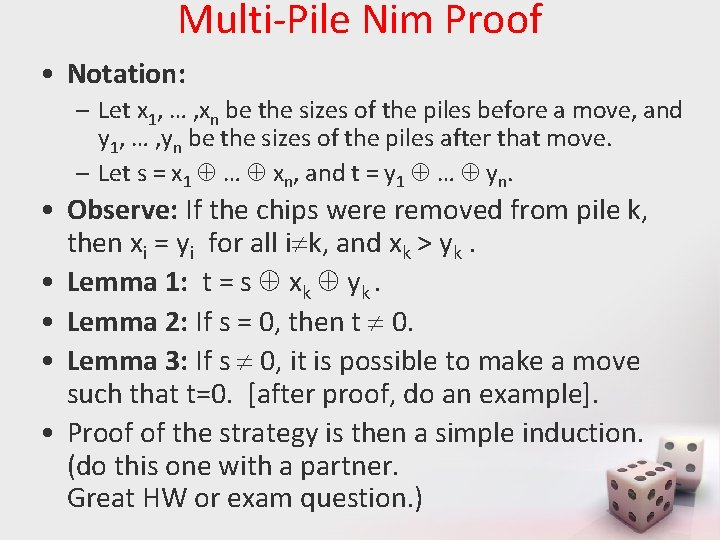Multi-Pile Nim Proof • Notation: – Let x 1, … , xn be the sizes of the piles before a move, and y 1, … , yn be the sizes of the piles after that move. – Let s = x 1 … xn, and t = y 1 … yn. • Observe: If the chips were removed from pile k, then xi = yi for all i k, and xk > yk. • Lemma 1: t = s xk yk. • Lemma 2: If s = 0, then t 0. • Lemma 3: If s 0, it is possible to make a move such that t=0. [after proof, do an example]. • Proof of the strategy is then a simple induction. (do this one with a partner. Great HW or exam question. )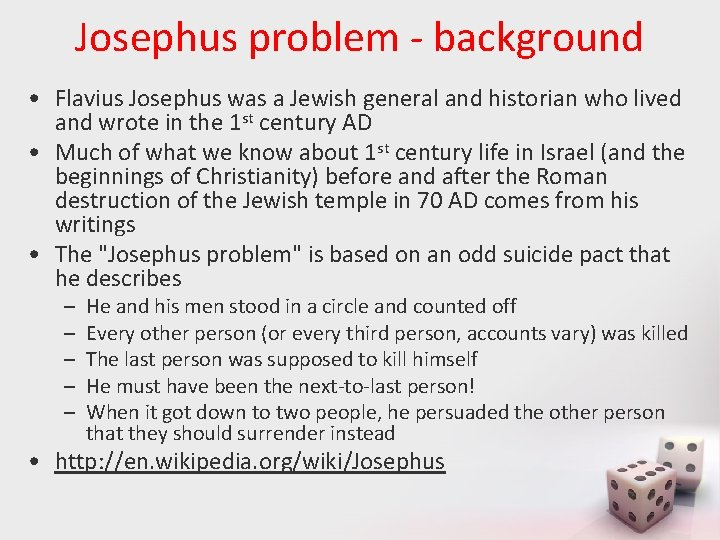Josephus problem - background • Flavius Josephus was a Jewish general and historian who lived and wrote in the 1 st century AD • Much of what we know about 1 st century life in Israel (and the beginnings of Christianity) before and after the Roman destruction of the Jewish temple in 70 AD comes from his writings • The "Josephus problem" is based on an odd suicide pact that he describes – – – He and his men stood in a circle and counted off Every other person (or every third person, accounts vary) was killed The last person was supposed to kill himself He must have been the next-to-last person! When it got down to two people, he persuaded the other person that they should surrender instead • http: //en. wikipedia. org/wiki/Josephus